# SpanBERT: Improving Pre-training by Representing and Predicting Spans

## SpanBERT

SpanBERT通过三种方式来帮助模型理解语言:

2. 引入一种新的辅助目标来SBO帮助模型训练.
3. 只使用单个句子训练, 而非BERT所使用的句子对.

$$\operatorname{enc}\left(x_{1}, x_{2}, \ldots, x_{n}\right)=\mathbf{x}_{1}, \mathbf{x}_{2}, \ldots, \mathbf{x}_{n}$$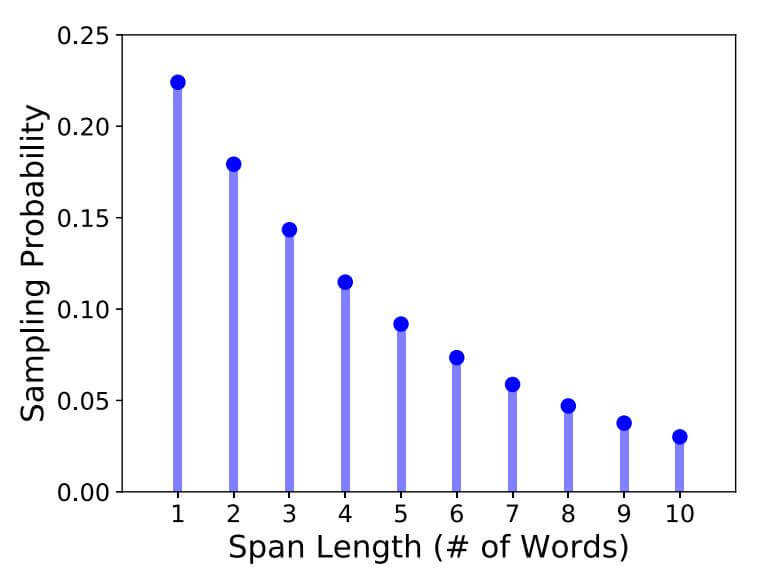\begin{aligned} &p = 0.2 \\ &q = 1 - p = 0.8 \\ &p^\prime = \frac{p}{1-q^{10}} = 0.224 \\ \end{aligned}
$p^\prime$ 代表Span最大长度为10以下的概率的归一化. $1-q^{10}$ 为当前Span不超过10的概率和, 需要用$p$ 去除以这个值, 让$p^\prime$ 排除掉Span长度大于10的情况.

\begin{aligned} E(x) &= p^\prime \sum_{n=1}^{10}n q^{n-1} \\ &= p^\prime (1 + 2q + 3q^2 + \dots + 10q^9) \\ &= 0.224 \cdot 16.9469=3.797 \approx3.8 \end{aligned}

### Span Boundary Objective

SBO的提出应该也受到一些其他模型的启发, 例如ERNIE(Baidu), BERT WWM等模型, 都是基于Span Level Mask做的额外处理.

$$\mathbf{y}_{i}=f\left(\mathbf{x}_{s-1}, \mathbf{x}_{e+1}, \mathbf{p}_{i-s+1}\right)$$

$$\begin{array}{l} \mathbf{h}_{0}=\left[\mathbf{x}_{s-1} ; \mathbf{x}_{e+1} ; \mathbf{p}_{i-s+1}\right] \\ \mathbf{h}_{1}=\text { LayerNorm }\left(\operatorname{GeLU}\left(\mathbf{W}_{1} \mathbf{h}_{0}\right)\right) \\ \mathbf{y}_{i}=\text { LayerNorm }\left(\operatorname{GeLU}\left(\mathbf{W}_{2} \mathbf{h}_{1}\right)\right) \end{array}$$

$[\cdot]$ 代表拼接操作.

SBO将和MLM的损失函数共同优化, 二者之间是简单的加和关系即可:
\begin{aligned} \mathcal{L}\left(x_{i}\right) &=\mathcal{L}_{\mathrm{MLM}}\left(x_{i}\right)+\mathcal{L}_{\mathrm{SBO}}\left(x_{i}\right) \\ &=-\log P\left(x_{i} \mid \mathbf{x}_{i}\right)-\log P\left(x_{i} \mid \mathbf{y}_{i}\right) \end{aligned}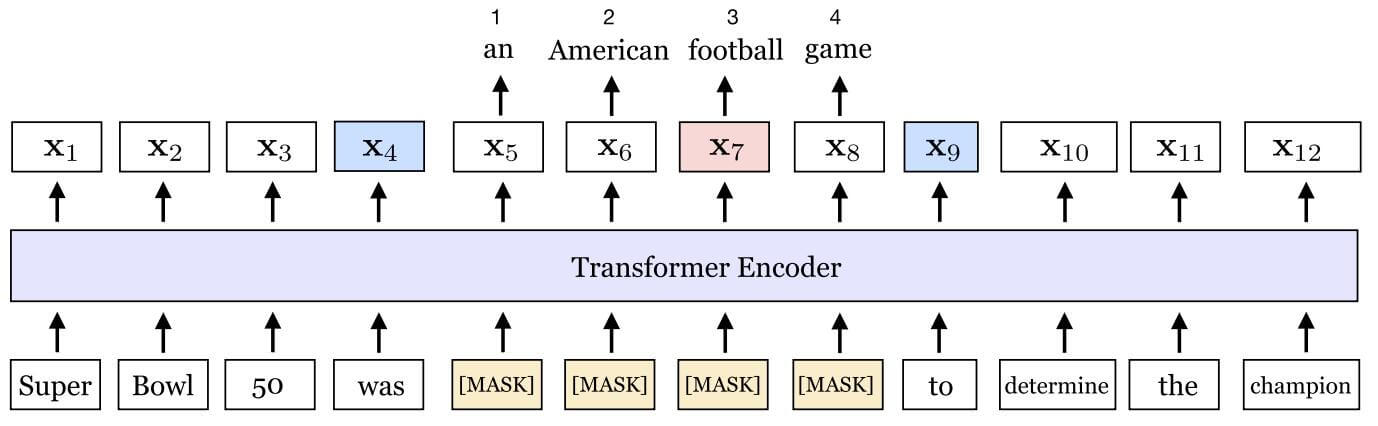\begin{aligned} \mathcal{L}(\text { football }) &=\mathcal{L}_{\mathrm{MLM}}(\text { football })+\mathcal{L}_{\mathrm{SBO}}(\text { football }) \\ &=-\log P\left(\text { football } \mid \mathbf{x}_{7}\right)-\log P\left(\text { football } \mid \mathbf{x}_{4}, \mathbf{x}_{9}, \mathbf{p}_{3}\right) \end{aligned}

### Single - Sequence Training

1. 句子对的引入限制了单句最长文本长度. 使用单句训练, 最长文本长度可以直接翻倍.
2. 句子对上下不相关时, 会引入非常大的噪声.

## Experiments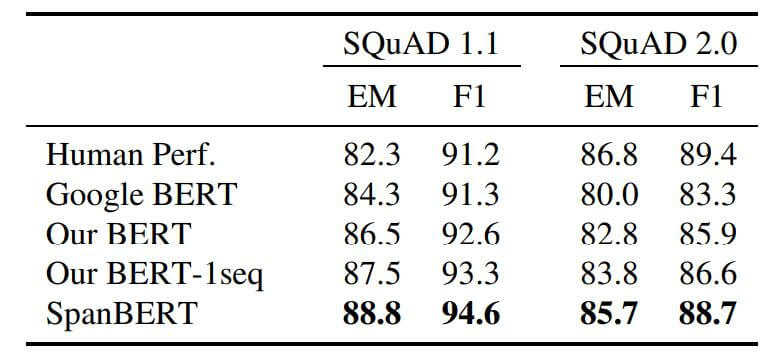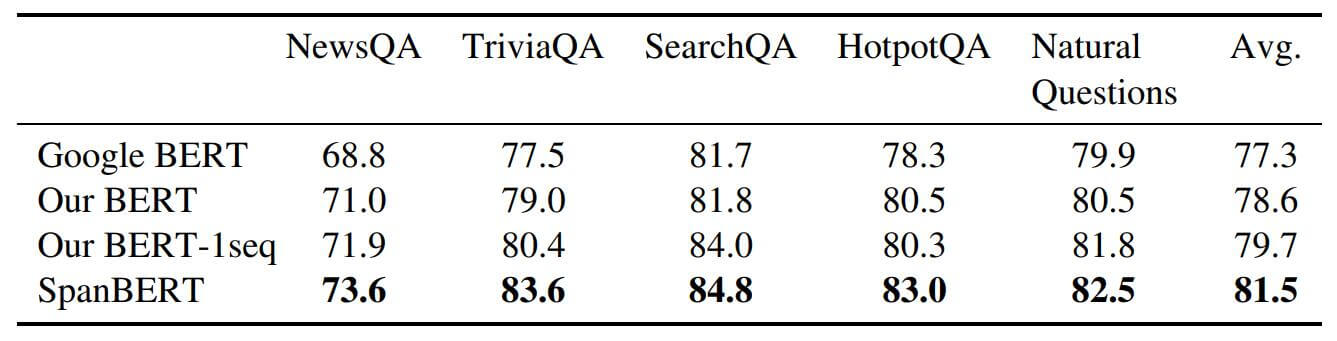### Coreference Resolution

$$P(y)=\frac{e^{s(x, y)}}{\sum_{y^{\prime} \in Y} e^{s\left(x, y^{\prime}\right)}}$$

\begin{aligned} s(x, y) &=s_{m}(x)+s_{m}(y)+s_{c}(x, y) \\ s_{m}(x) &=\mathrm{FFNN}_{m}\left(\mathbf{g}_{\mathrm{x}}\right) \\ s_{c}(x, y) &=\mathrm{FFNN}_{c}\left(\mathbf{g}_{\mathrm{x}}, \mathbf{g}_{\mathbf{y}}, \phi(x, y)\right) \end{aligned}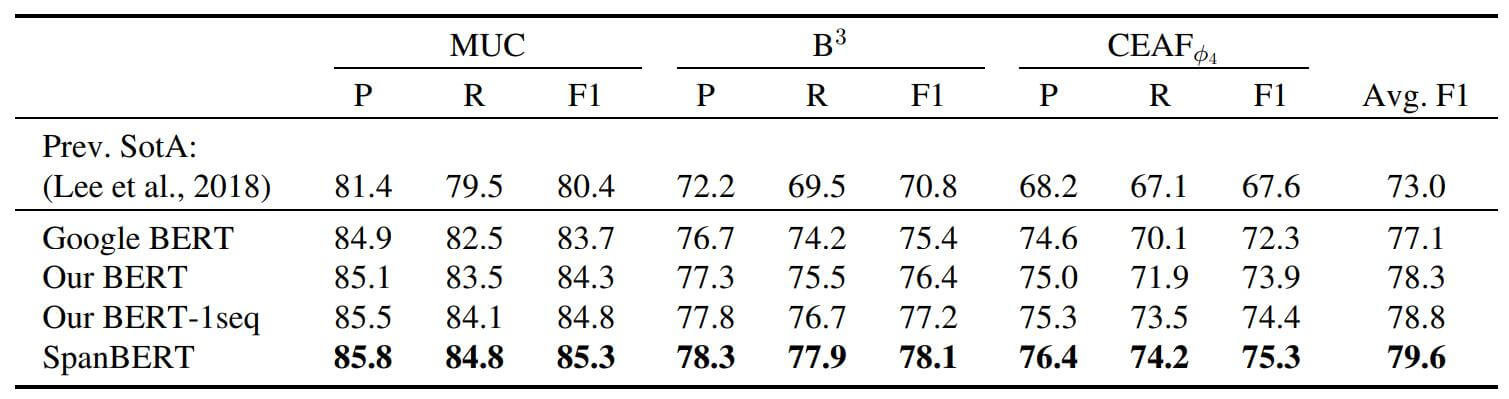### Relation Extraction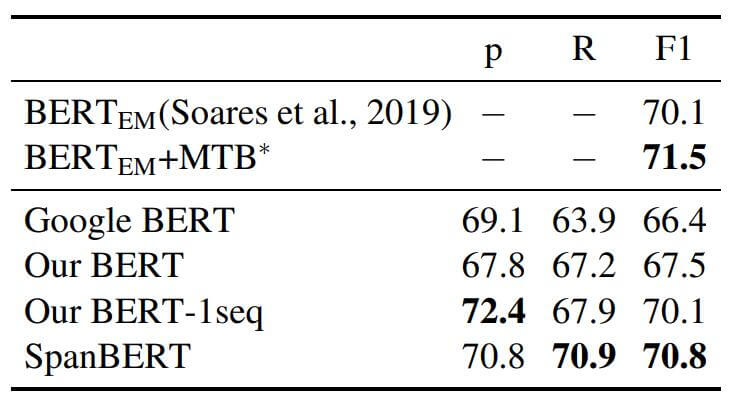SpanBERT在关系抽取上的标现达到平均水平, 在Recall上进步比较大.

### GLUE

GLUE上的结果如下: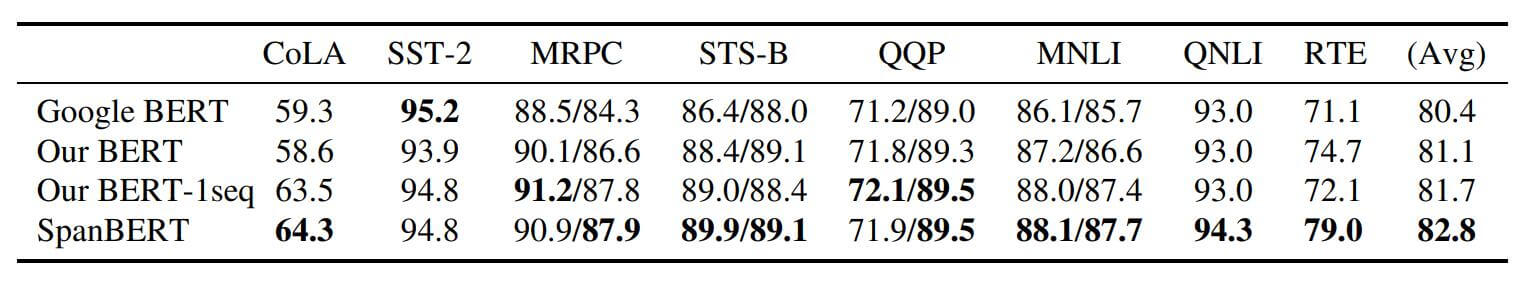### Ablation Study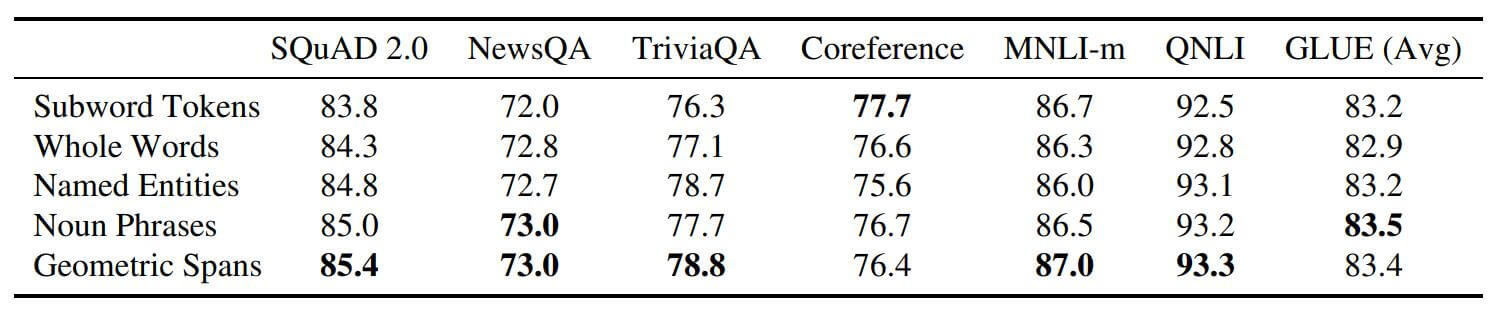#### Auxiliary Objectives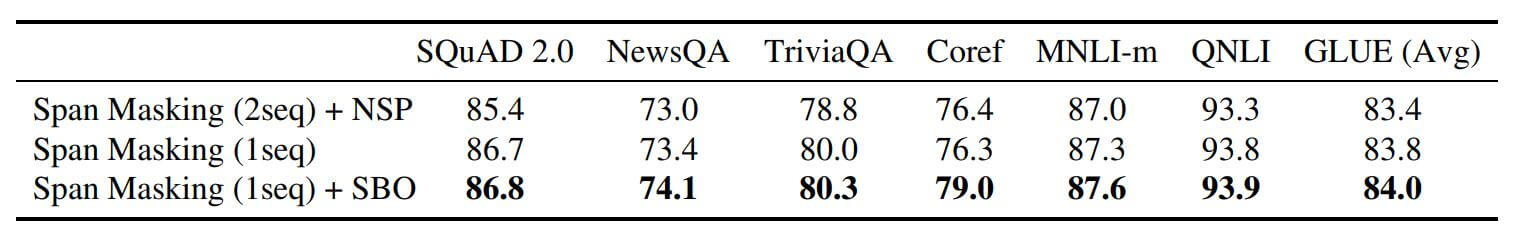## Summary

SpanBERT在各类任务上性能皆超过BERT, 应该算作是一种比较有效的改进方式, 方法也比较巧妙.

上一篇MASS: Masked Sequence to Sequence Pre - training for Language Generation

2021-06-08

目录